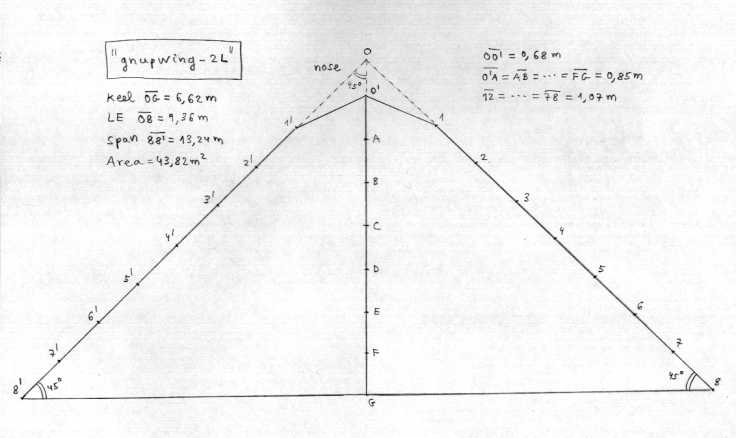gnupwing-2L

The gnupwing-2L is a wing parawing of two lobules. The planform and the length of lines for a model of 43,28 m2 are indicated.

The lengths of lines have been obtained from a model at scale and still need optimization about a new model. The length of lines indicated can shorten in 1,2 m, obtaining the lengths of anchorage to the maillons from the four bands.

The edges of fabric 1' -0' -1 they have to withdraw about themselves formant one near attack rounded like in the gnupwing of three lobules.

 gnupwing-2L Basic parameters: Keel= 6,62 m LE= 9,36 m span= 13,24 m Area= 43,82 m2 Planform: 0'O= 0,68 0'1= 1,49 OA= 0,85 12= 1,07 AB= 0,85 23= 1,07 BC= 0,85 34= 1,07 CD= 0,85 45= 1,07 DE= 0,85 56= 1,07 EF= 0,85 67= 1,07 FG= 0,85 78= 1,07 OG= 5,95 18= 7,47 Lines: Keel LE O= 10,8 1= 10,8 A= 10,98 2= 10,73 B= 11,14 3= 10,6 C= 11,25 4= 10,4 D= 11,25 5= 10,15 E= 11,22 6= 9,72 F= 11,01 7= 9,04 G= 10,8 8= 8,37index